# Continuity & Differentiability, NCERT Solutions, Class 12, Maths , Part -8 Notes - JEE

## JEE: Continuity & Differentiability, NCERT Solutions, Class 12, Maths , Part -8 Notes - JEE

The document Continuity & Differentiability, NCERT Solutions, Class 12, Maths , Part -8 Notes - JEE is a part of JEE category.
All you need of JEE at this link: JEE

Question 1:
Verify Rolle’s Theorem for the function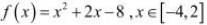The given function,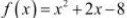, being a polynomial function, is continuous in [−4,2] and is differentiable in (−4, 2).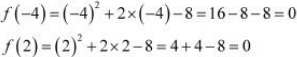∴ f (−4) = f (2) = 0
⇒ The value of f (x) at −4 and 2 coincides.
Rolle’s Theorem states that there is a point c ∈ (−4, 2) such that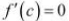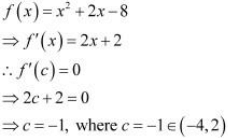Hence, Rolle’s Theorem is verified for the given function.

Question 2:
Examine if Rolle’s Theorem is applicable to any of the following functions. Can you say
some thing about the converse of Rolle’s Theorem from these examples?
(i)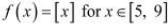(ii)(iii)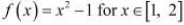By Rolle’s Theorem, for a function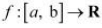, if

(a) f is continuous on [a, b]
(b) f is differentiable on (a, b)
f (a) = f (b)
then, there exists some c ∈ (a, b) such that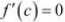Therefore, Rolle’s Theorem is not applicable to those functions that do not satisfy any of
the three conditions of the hypothesis.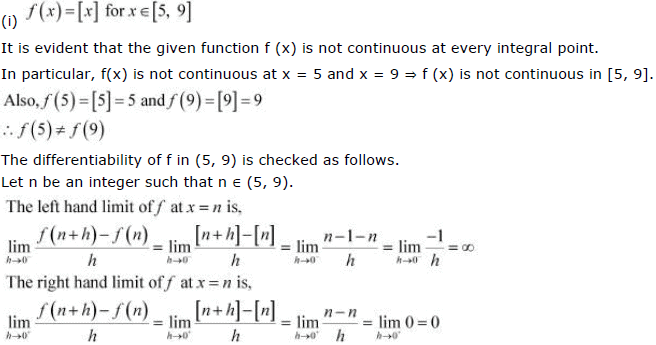Since the left and right hand limits of f at x = n are not equal, f is not differentiable at x = n
∴ f is not differentiable in (5, 9).
It is observed that f does not satisfy all the conditions of the hypothesis of Rolle’s Theorem.
Hence, Rolle’s Theorem is not applicable for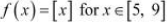.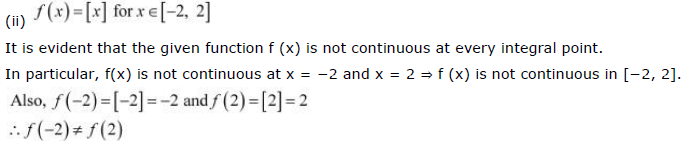The differentiability of f in (−2, 2) is checked as follows.
Let n be an integer such that n ∈ (−2, 2).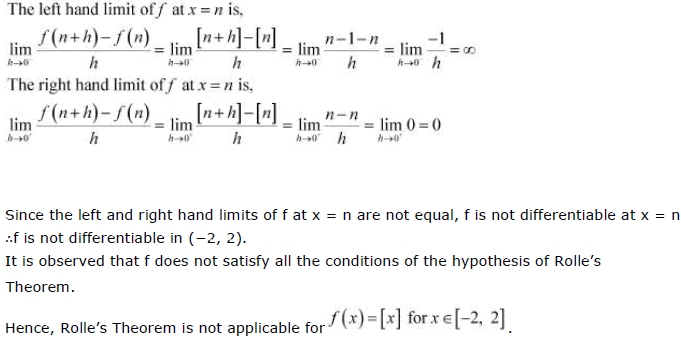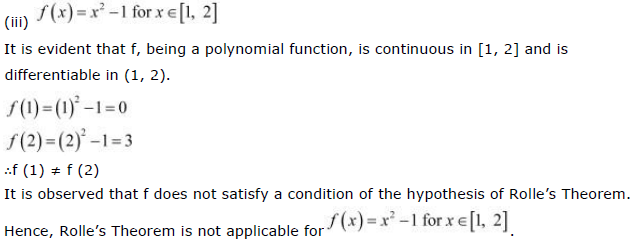Question 3:
If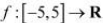is a differentiable function and if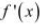does not vanish anywhere,

then prove that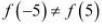It is given thatis a differentiable function.
Since every differentiable function is a continuous function, we obtain
(a) f is continuous on [−5, 5].
(b) f is differentiable on (−5, 5).
Therefore, by the Mean Value Theorem, there exists c ∈ (−5, 5) such that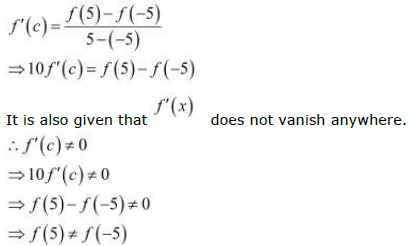Hence, proved.

Question 4:
Verify Mean Value Theorem, if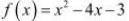in the interval [a, b], where a =1 and b = 3.

The given function isf, being a polynomial function, is continuous in [1, 4] and is differentiable in (1, 4) whose derivative is 2x − 4.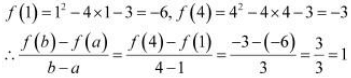Mean Value Theorem states that there is a point c (1, 4) such that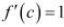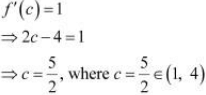Hence, Mean Value Theorem is verified for the given function.

Question 5:

Verify Mean Value Theorem , if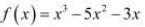in the interval [a, b], where a =1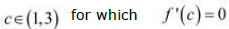b=3
Find all c

The given function f is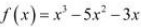f, being a polynomial function, is continuous in [1, 3] and is differentiable in (1, 3)
whose derivative is 3x2 − 10x − 3.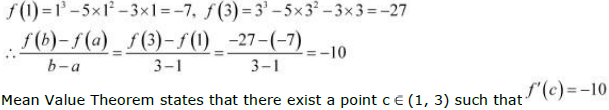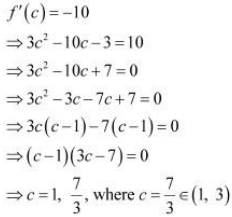Hence, Mean Value Theorem is verified for the given function and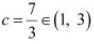is the
only point for which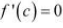Question 6:
Examine the applicability of Mean Value Theorem for all three functions given in the above exercise 2.

Mean Value Theorem states that for a function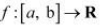, if
(a) f is continuous on [a, b]
(b) f is differentiable on (a, b)
then, there exists some c ∈ (a, b) such that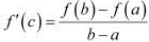Therefore, Mean Value Theorem is not applicable to those functions that do not satisfy
any of the two conditions of the hypothesis.

(i)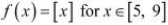It is evident that the given function f (x) is not continuous at every integral point.
In particular, f(x) is not continuous at x = 5 and x = 9 ⇒ f (x) is not continuous in [5, 9].
The differentiability of f in (5, 9) is checked as follows.
Let n be an integer such that n ∈ (5, 9).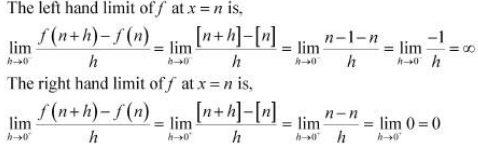Since the left and right hand limits of f at x = n are not equal, f is not differentiable at x = n
∴ f is not differentiable in (5, 9).
It is observed that f does not satisfy all the conditions of the hypothesis of Mean Value Theorem.
Hence, Mean Value Theorem is not applicable for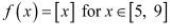.

(ii)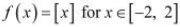It is evident that the given function f (x) is not continuous at every integral point.
In particular, f(x) is not continuous at x = −2 and x = 2 ⇒ f (x) is not continuous in [−2, 2].
The differentiability of f in (−2, 2) is checked as follows.
Let n be an integer such that n ∈ (−2, 2).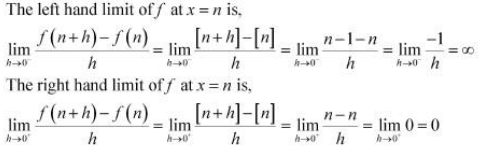Since the left and right hand limits of f at x = n are not equal, f is not differentiable at x = n
∴ f is not differentiable in (−2, 2).
It is observed that f does not satisfy all the conditions of the hypothesis of Mean Value Theorem.
Hence, Mean Value Theorem is not applicable for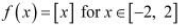.

(iii)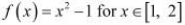It is evident that f, being a polynomial function, is continuous in [1, 2] and is differentiable in (1, 2).
It is observed that f satisfies all the conditions of the hypothesis of Mean Value Theorem.
Hence, Mean Value Theorem is applicable for.
It can be proved as follows.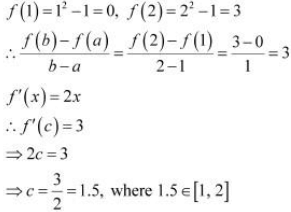The document Continuity & Differentiability, NCERT Solutions, Class 12, Maths , Part -8 Notes - JEE is a part of JEE category.
All you need of JEE at this link: JEEUse Code STAYHOME200 and get INR 200 additional OFF

Track your progress, build streaks, highlight & save important lessons and more!

,

,

,

,

,

,

,

,

,

,

,

,

,

,

,

,

,

,

,

,

,

,

,

,

,

,

,

,

,

,

,

,

,

;